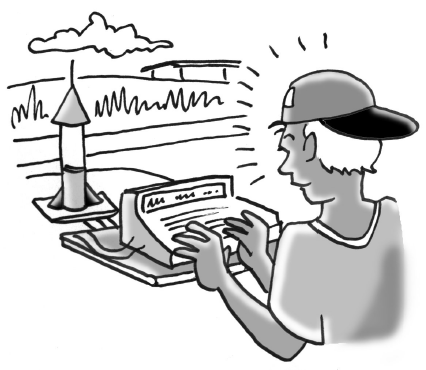### Home > INT2 > Chapter 9 > Lesson 9.3.4 > Problem9-121

9-121.

Chad is entering a rocket competition.1. Chad programs his rocket to launch straight up into the air with the function $h(t) = -16t^2 + 92t$, where $h$ represents the height in feet, and $t$ represents the time in seconds. How long after the launch does the rocket reach the ground?

When it hits the ground, what is the height?

When $h = 0$, $t = 5.75$ seconds.

2. Write and solve an inequality that shows when (during what time period) the rocket is at least $76$ feet high.

$-16t^2 + 92t ≥ 76$

$1 ≤ t ≤ 4.75$ seconds

3. In order to qualify, the rocket must be at least $76$ feet off the ground for four seconds. Does Chad’s rocket qualify?

For how many seconds is the rocket above $76$ feet.

4. What is an appropriate domain for the function in this situation?

For what times is the height greater than or equal to zero?